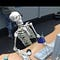Finding the Largest Prime Factor in Ruby

def is_prime?(n)
tests = Array (2..Math.sqrt(n).floor)
tests = tests.select { |x| n % x == 0}
return true if tests.empty?
false
end
def largest_prime_factor(n)
test = 3
out = 0
while test <= n
if ((n % test == 0) && is_prime?(test))
out = test
end
test+=1
end
return out
endPoor sucker’s coworkers didn’t even notice.
n = n/test
def largest_prime_factor(n)
test = 3
out = 0
while test <= n
if ((n % test == 0) && is_prime?(test))
out = test
n = n/test
end
test+=1
end
return out
end
Welcome to a place where words matter. On Medium, smart voices and original ideas take center stage - with no ads in sight. Watch
Follow all the topics you care about, and we’ll deliver the best stories for you to your homepage and inbox. Explore
Get unlimited access to the best stories on Medium — and support writers while you’re at it. Just \$5/month. Upgrade